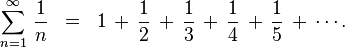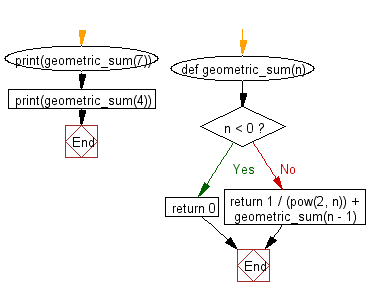﻿ Python Data Structures and Algorithms - Calculate the geometric sum - w3resource# Python Data Structures and Algorithms - Recursion: Calculate the geometric sum

## Python Recursion: Exercise-9 with Solution

Write a Python program to calculate the geometric sum of n-1.
Note : In mathematics, a geometric series is a series with a constant ratio between successive terms.

Example :Sample Solution:-

Python Code:

``````def geometric_sum(n):
if n < 0:
return 0
else:
return 1 / (pow(2, n)) + geometric_sum(n - 1)

print(geometric_sum(7))
print(geometric_sum(4))
```
```

Sample Output:

```1.9921875
1.9375
```

Flowchart:## Visualize Python code execution:

The following tool visualize what the computer is doing step-by-step as it executes the said program:

Python Code Editor:

What is the difficulty level of this exercise?

Test your Programming skills with w3resource's quiz.

﻿

## Python: Tips of the Day

Decapitalizes the first letter of a string:

Example:

```def tips_decapitalize(s, upper_rest=False):
return s[:1].lower() + (s[1:].upper() if upper_rest else s[1:])
print(tips_decapitalize('PythonTips'))
print(tips_decapitalize('PythonTips', True))
```

Output:

```pythonTips
pYTHONTIPS
```